We’d like to understand how you use our websites in order to improve them. Register your interest.

# Existence of positive solutions of elliptic mixed boundary value problem

## Abstract

In this paper, we use variational methods to prove two existence of positive solutions of the following mixed boundary value problem:

${ − Δ u = f ( x , u ) , x ∈ Ω , u = 0 , x ∈ σ , ∂ u ∂ ν = g ( x , u ) , x ∈ Γ .$

One deals with the asymptotic behaviors of $f(x,u)$ near zero and infinity and the other deals with superlinear of $f(x,u)$ at infinity.

MSC:35M12, 35D30.

## 1 Introduction and preliminaries

This paper is concerned with the existence of positive solutions of the following elliptic mixed boundary value problem:

${ − Δ u = f ( x , u ) , x ∈ Ω , u = 0 , x ∈ σ , ∂ u ∂ ν = g ( x , u ) , x ∈ Γ ,$
(1)

where Ω is a bounded domain in $R n$ with Lipschitz boundary Ω, $σ∪Γ=∂Ω$, $σ∩Γ=Ø$, Γ is a sufficiently smooth $(n−1)$-dimensional manifold, and ν is the outward normal vector on Ω. We assume $f:Ω×R→R$, $g:Γ×R→R$ are continuous and satisfy

(S1) $f(x,t)≥0$, $∀t≥0$, $x∈Ω$, $f(x,0)=0$. $f(x,t)≡0$, $∀t<0$, $x∈Ω$.

(S2) For almost every $x∈Ω$, $f ( x , t ) t$ is nondecreasing with respect to $t>0$.

(S3) $lim t → 0 f ( x , t ) t =p(x)$, $lim t → + ∞ f ( x , t ) t =q(x)≢0$ uniformly in a.e. $x∈Ω$, where $∥ p ( x ) ∥ ∞ < λ 1$, $λ 1$ is the first eigenvalue of (2), $0≤p(x)$, $q(x)∈ L ∞ (Ω)$.

(S4) There exists $c 1 , c 2 >0$ such that $|f(x,t)|≤ c 1 + c 2 | t | p − 1$ for some $p∈(2, 2 n n − 2 )$ as $n≥3$ and $p∈(2,+∞)$ as $n=1,2$.

The eigenvalue problem of (1) is studied by Liu and Su in 

(2)

There exists a set of eigenvalues ${ λ k }$ and corresponding eigenfunctions ${ u k }$ which solve problem (2), where $0≤ λ 1 ≤ λ 2 ≤⋯≤ λ k ≤⋯$, $λ k →∞$ as $k→∞$, $λ 1 = inf 0 ≠ u ∈ V ∫ Ω | ∇ u | 2 d x ∫ Ω | u | 2 d x + ∫ Γ | u | 2 d s$.

There have been many papers concerned with similar problems at resonance under the boundary condition; see . Moreover, some multiplicity theorems are obtained by the topological degree technique and variational methods; interested readers can see . Problem (1) is different from the classical ones, such as those with Dirichlet, Neuman, Robin, No-flux, or Steklov boundary conditions.

In this paper, we assume $V:={v∈ H 1 (Ω):v | σ =0}$ is a closed subspace of $H 1 (Ω)$. We define the norm in V as $∥ u ∥ 2 = ∫ Ω | ∇ u | 2 dx+ ∫ Γ | γ u | 2 ds$, $∥ ⋅ ∥ L p ( Ω )$ is the $L p (Ω)$ norm, $∥ ⋅ ∥ L p ( Γ )$ is the $L p (Γ)$ norm, $γ:V→ L 2 (Γ)$ is the trace operator with $γu= u Γ$ for all $u∈ H 1 (Ω)$, that is continuous and compact (see ). Furthermore, we define $g=γf$, $0≤g(x,t)≤|γf(x,t)|$ for $t>0$ (see ). Then, by (S3), we obtain

(3)

Let Ω be a bounded domain with a Lipschitz boundary; there is a continuous embedding $V↪ L y (Ω)$ for $y∈[2, 2 n n − 2 ]$ when $n≥3$, and $y∈[2,+∞)$ when $n=1,2$. Then there exists $γ y >0$, such that

$∥ u ∥ L y ( Ω ) ≤ γ y ∥u∥,∀u∈V.$
(4)

Moreover, there is a continuous boundary trace embedding $V↪ L z (Γ)$ for $z∈[2, 2 ( n − 1 ) n − 2 ]$ when $n≥3$, and $z∈[2,+∞)$ when $n=1,2$. Then there exists $k z >0$, such that

$∥ u ∥ L z ( Γ ) ≤ k z ∥u∥,∀u∈V.$
(5)

It is well known that to seek a nontrivial weak solution of problem (1) is equivalent to finding a nonzero critical value of the $C 1$ functional

$J(u)= 1 2 ∫ Ω | ∇ u | 2 dx− ∫ Ω F(x,u)dx− ∫ Γ G(s,u)ds,$
(6)

where $u∈V$, $F(x,u)= ∫ 0 u f(x,t)dt$, $G(x,u)= ∫ 0 u g(x,t)dt$. Moreover, by (S1) and the Strong maximum principle, a nonzero critical point of J is in fact a positive solution of (1). In order to find critical points of the functional (6), one often requires the technique condition, that is, for some $μ>2$, $∀|u|≥M>0$, $x∈Ω$,

$0<μF(x,u)≤uf(x,u),F(x,u)= ∫ 0 u f(x,t)dt.$
(AR)

It is easy to see that the condition (AR) implies that $lim u → + ∞ F ( x , u ) u 2 =+∞$, that is, $f(x,u)$ must be superlinear with respect to u at infinity. In the present paper, motivated by  and , we study the existence and nonexistence of positive solutions for problem (1) with the asymptotic behavior assumptions (S3) of f at zero and infinity. Moreover, we also study superlinear of f at infinity with $q(x)≡+∞$ in (S3), which is weaker than the (AR) condition, that is the (AR) condition does not hold.

In order to get our conclusion, we define the minimization problem

$Λ=inf { ∫ Ω | ∇ u | 2 d x : u ∈ V , ∫ Ω q ( x ) u 2 d x + ∫ Γ q ( s ) u 2 d s = 1 } ,$
(7)

then $Λ>0$, which is achieved by some $φ Λ ∈V$ with $φ Λ (x)>0$ a.e. in Ω; see Lemma 1.

We denote by c, $c 1$, $c 2$ universal constants unless specified otherwise. Our main results are as follows.

Theorem 1 Let conditions (S 1) to (S 3) hold, then:

1. (i)

If $Λ>1$, then the problem (1) has no any positive solution in V.

2. (ii)

If $Λ<1$, then the problem (1) has at least one positive solution in V.

3. (iii)

If $Λ=1$, then the problem (1) has one positive solution $u(x)∈V$ if and only if there exists a constant $c>0$ such that $u(x)=c φ Λ (x)$ and $f(x,u)=q(x)u(x)$, $g(x,u)=q(x)u(x)$ a.e. $x∈Ω$, where $φ Λ (x)>0$ is the function which achieves Λ.

Corollary 2 Let conditions (S 1) to (S 3) with $q(x)≡l>0$ hold, then:

1. (i)

If $l< λ 1$, then the problem (1) has no any positive solution in V.

2. (ii)

If $λ 1 , then the problem (1) has at least one positive solution in V.

3. (iii)

If $l= λ 1$, then the problem (1) has one positive solution $u(x)∈V$ if and only if there exists a constant $c>0$ such that $u(x)=c φ 1 (x)$ and $f(x,u)= λ 1 u(x)$, $g(x,u)= λ 1 u(x)$ a.e. $x∈Ω$, where $φ 1 (x)>0$ is the eigenfunction of the $λ 1$.

Theorem 3 Let conditions (S 1) to (S 4) with $q(x)≡+∞$ hold, then the problem (1) has at least one positive solution in V.

## 2 Some lemmas

We need the following lemmas.

Lemma 1 If $q(x)∈ L ∞ (Ω)$, $q(x)≥0$, $q(x)≢0$, then $Λ>0$ and there exists $φ Λ (x)∈V$ such that $Λ= ∫ Ω | ∇ φ Λ | 2 dx$ and $∫ Ω q(x) φ Λ 2 dx+ ∫ Γ q(s) φ Λ 2 ds=1$. Moreover, $φ Λ (x)>0$ a.e. in V.

Proof By the Sobolev embedding function $V↪ L 2 (Ω)$ and Fatou’s lemma, it is easy to know that $Λ>0$ and there exists $φ Λ (x)∈V$, which satisfies Λ, that is, $∫ Ω q(x) φ Λ 2 dx+ ∫ Γ q(s) φ Λ 2 ds=1$. Furthermore, we assume $φ Λ (x)≥0$, then $φ Λ (x)$ could replace by $| φ Λ (x)|$. By the Strong maximum principle, we know $φ Λ (x)>0$ a.e. in V. □

Lemma 2 If conditions (S 1) to (S 3) hold, then there exists $β,ρ>0$ such that $J | ∂ B ρ ( 0 ) ≥β$, $∀u∈V$, $∥u∥=ρ$.

Proof By condition (S3), there exists $δ>0$, $ε>0$ such that $f ( x , u ) u ≤ λ 1 −ε$, $g ( x , u ) u ≤ γ f ( x , u ) u ≤ λ 1 −ε$ as $0<|u|≤δ$. Which implies that $F(x,u)≤ 1 2 ( λ 1 −ε) u 2 +c | u | y$, $G(x,u)≤ 1 2 ( λ 1 −ε) u 2 +c | u | z$.

By (4) and (5), we obtain

$J ( u ) = 1 2 ∥ ∇ u ∥ L 2 ( Ω ) 2 − ∫ Ω F ( x , u ) d x − ∫ Γ G ( s , u ) d s ≥ 1 2 ∥ ∇ u ∥ L 2 ( Ω ) 2 + 1 2 ∥ γ u ∥ L 2 ( Γ ) 2 − 1 2 ∥ γ u ∥ L 2 ( Γ ) 2 − 1 2 ( λ 1 − ε ) ∥ u ∥ L 2 ( Ω ) 2 − c ∥ u ∥ L y ( Ω ) y − 1 2 ( λ 1 − ε ) ∥ u ∥ L 2 ( Γ ) 2 − c ∥ u ∥ L z ( Γ ) z ≥ 1 2 ∥ u ∥ 2 − 1 2 ( λ 1 − ε ) 1 λ 1 ∥ u ∥ 2 − c γ y y ∥ u ∥ y − 1 2 ( λ 1 − ε + 1 ) 1 λ 1 + 1 ∥ u ∥ 2 − c k z z ∥ u ∥ z = [ ε ( 2 λ 1 + 1 ) 2 λ 1 ( λ 1 + 1 ) − 1 2 ] ∥ u ∥ 2 − c γ y y ∥ u ∥ y − c k z z ∥ u ∥ z .$

Hence, $y,z>2$; we take ε which satisfies $ε ( 2 λ 1 + 1 ) 2 λ 1 ( λ 1 + 1 ) − 1 2 >0$, that is, $ε> λ 1 ( λ 1 + 1 ) 2 λ 1 + 1$. Then we take a positive constant β such that $J | ∂ B ρ ( 0 ) ≥β$ as $∥u∥=ρ$, and is small enough. □

Lemma 3 If conditions (S 1) to (S 3) hold, $Λ<1$, $φ Λ (x)>0$ is defined by Lemma 1, then $J(t φ Λ (x))→−∞$ as $t→+∞$.

Proof If $Λ<1$, $φ Λ (x)>0$ is defined by Lemma 1, by Fatou’s lemma, and (S3), we have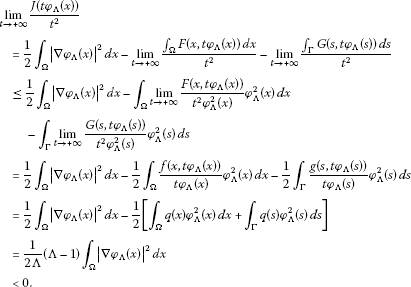So, $J(t φ Λ (x))→−∞$ as $t→+∞$. □

Lemma 4 Let conditions (S 1) and (S 2) hold. If a sequence ${ u n }⊂V$ satisfies $〈 J ′ ( u n ), u n 〉→0$ as $n→+∞$, then there exists a subsequence of ${ u n }$, still denoted by ${ u n }$ such that $J(t u n )≤ 1 + t 2 2 n +J( u n )$ for all $t>0$, $n≥1$.

Proof Since $〈 J ′ ( u n ), u n 〉→0$ as $n→+∞$, for a subsequence, we may assume that

$− 1 n < 〈 J ′ ( u n ) , u n 〉 = ∥ ∇ u n ∥ L 2 ( Ω ) 2 − ∫ Ω f(x, u n ) u n dx− ∫ Γ g(s, u n ) u n ds< 1 n ,∀n≥1.$
(8)

For any fixed $x∈Ω$ and $n≥1$, set

$ψ 1 (t)= t 2 2 f(x, u n ) u n −F(x,t u n ), ψ 2 (t)= t 2 2 g(s, u n ) u n −G(s,t u n ).$

Then (S2) implies that

$ψ 1 ′ ( t ) = t f ( x , u n ) u n − f ( x , t u n ) u n = t u n [ f ( x , u n ) − f ( x , t u n ) t ] = { ≥ 0 , 0 < t ≤ 1 ; ≤ 0 , t > 1 .$

It implies that $ψ 1 (t)≤ ψ 1 (1)$, $∀t>0$. Following the same procedures, we obtain $ψ 2 (t)≤ ψ 2 (1)$, $∀t>0$.

For all $t>0$ and positive integer n, by (8), we have

$J ( t u n ) = t 2 2 ∥ ∇ u n ∥ L 2 ( Ω ) 2 − ∫ Ω F ( x , t u n ) d x − ∫ Γ G ( s , t u n ) d s ≤ t 2 2 [ 1 n + ∫ Ω f ( x , u n ) u n d x + ∫ Γ g ( s , u n ) u n d s ] − ∫ Ω F ( x , t u n ) d x − ∫ Γ G ( s , t u n ) d s ≤ t 2 2 n + ∫ Ω [ 1 2 f ( x , u n ) u n − F ( x , u n ) ] d x + ∫ Γ [ 1 2 g ( s , u n ) u n − G ( s , u n ) ] d s .$
(9)

On the other hand, by (8), one has

$J ( u n ) = 1 2 ∥ ∇ u n ∥ L 2 ( Ω ) 2 − ∫ Ω F ( x , u n ) d x − ∫ Γ G ( s , u n ) d s ≥ 1 2 [ − 1 n + ∫ Ω f ( x , u n ) u n d x + ∫ Γ g ( s , u n ) u n d s ] − ∫ Ω F ( x , u n ) d x − ∫ Γ G ( s , u n ) d s = − 1 2 n + ∫ Ω [ 1 2 f ( x , u n ) u n − F ( x , u n ) ] d x + ∫ Γ [ 1 2 g ( s , u n ) u n − G ( s , u n ) ] d s .$

One has

$∫ Ω [ 1 2 f ( x , u n ) u n − F ( x , u n ) ] dx+ ∫ Γ [ 1 2 g ( s , u n ) u n − G ( s , u n ) ] ds≤J( u n )+ 1 2 n .$
(10)

Combining (9) and (10), we have $J(t u n )≤ 1 + t 2 2 n +J( u n )$. □

Lemma 5 (see )

Suppose E is a real Banach space, $J∈ C 1 (E,R)$ satisfies the following geometrical conditions:

1. (i)

$J(0)=0$; there exists $ρ>0$ such that $J | ∂ B ρ ( 0 ) ≥r>0$;

2. (ii)

There exists $e∈E∖ B ρ ( 0 ) ¯$ such that $J(e)≤0$. Let $Γ 1$ be the set of all continuous paths joining 0 and e:

$Γ 1 = { h ∈ C ( [ 0 , 1 ] , E ) | h ( 0 ) = 0 , h ( 1 ) = e } ,$

and

$c= inf h ∈ Γ 1 max t ∈ [ 0 , 1 ] J ( h ( t ) ) .$

Then there exists a sequence ${ u n }⊂E$ such that $J( u n )→c≥β$ and $(1+∥ u n ∥)× ∥ J ′ ( u n ) ∥ E ∗ →0$.

## 3 Proofs of main results

Proof of Theorem 1 (i) If $u∈V$ is one positive solution of problem (1), by (3), one has

$0= 〈 J ′ ( u ) , u 〉 = ∫ Ω | ∇ u | 2 dx− ∫ Ω f(x,u)udx− ∫ Γ g(s,u)uds.$

That is,

$∫ Ω | ∇ u | 2 d x = ∫ Ω f ( x , u ) u d x + ∫ Γ g ( s , u ) u d s ≤ ∫ Ω q ( x ) u 2 d x + ∫ Γ q ( s ) u 2 d s = 1 .$

It implies that $Λ≤1$. This completes the proof of Theorem 1(i).

1. (ii)

By Lemma 2, there exists $β,ρ>0$ such that $J | ∂ B ρ ( 0 ) ≥β$ with $∥u∥=ρ$. By Lemma 3, we obtain $J( t 0 φ Λ (x))<0$ as $t 0 →+∞$. Define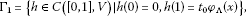(11)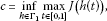(12)

where $φ Λ (x)>0$ is given by Lemma 1. Then $c≥β>0$ and by Lemma 3, there exists ${ u n }⊂V$ such that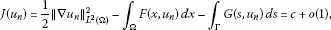(13)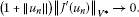(14)
1. (14)

implies that

$〈 J ′ ( u n ) , u n 〉 = ∥ ∇ u n ∥ L 2 ( Ω ) 2 − ∫ Ω f(x, u n ) u n dx− ∫ Γ g(s, u n ) u n ds=o(1).$
(15)

Here, in what follows, we use $o(1)$ to denote any quantity which tends to zero as $n→+∞$.

If ${ u n }$ is bounded in V, when Ω is bounded and $f(x,u)$, $g(x,u)$ are subcritical, we can get ${ u n }$ has a subsequence strong convergence to a critical value of J, and our proof is complete. So, to prove the theorem, we only need show that ${ u n }$ is bounded in V. Supposing that ${ u n }$ is unbounded, that is, $∥ u n ∥→+∞$ as $n→+∞$. We order

$t n = 2 c ∥ u n ∥ , w n = t n u n = 2 c u n ∥ u n ∥ .$
(16)

Then ${ w n }$ is bounded in V. By extracting a subsequence, we suppose $w n →w$ is a strong convergence in $L 2 (Ω)$, $w n →w$ is a convergence a.e. $x∈Ω$, $w n ⇀w$ is a weak convergence in V.

We claim that $w≠0$. In fact, by (S1) and (S3), we know $∀x∈Ω$, $u n ≥0$, and there exists $M 1 , M 2 >0$ such that $| f ( x , u n ) u n |≤ M 1$, $| g ( x , u n ) u n |≤ M 2$. If $w=0$, $w n →0$ is a strong convergence in $L 2 (Ω)$, and by (15) and (16) we know

$4 c = t n 2 ∥ u n ∥ 2 = t n 2 ( ∥ ∇ u n ∥ L 2 ( Ω ) 2 + ∥ γ u n ∥ L 2 ( Γ ) 2 ) = t n 2 ∫ Ω f ( x , u n ) u n d x + t n 2 ∫ Γ g ( s , u n ) u n d s + t n 2 ∥ γ u n ∥ L 2 ( Γ ) 2 + o ( 1 ) = ∫ Ω f ( x , u n ) u n w n 2 d x + ∫ Γ g ( s , u n ) u n w n 2 d s + t n 2 ∥ u n ∥ L 2 ( Γ ) 2 + o ( 1 ) ≤ M 1 ∫ Ω w n 2 d x + M 2 ∫ Γ w n 2 d s + ∥ w n ∥ L 2 ( Γ ) 2 + o ( 1 ) → 0 .$

It is contradiction with $c>0$, so $w≠0$.

As follows, we prove $w≠0$ satisfies

$∫ Ω ∇φ(x)∇w(x)dx− ∫ Ω q 1 (x)φ(x)w(x)dx− ∫ Γ q 2 (s)φ(s)w(s)ds=0.$

We order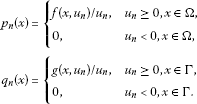By (S1) and (S3), there exists $M 3 >0$ such that $0≤ p n (x)≤ M 3$, $0≤ q n (x)≤ M 3$, $∀x∈ Ω ¯$. We select a suitable subsequence and there exists $h 1 (x)∈ L 2 (Ω)$, $h 2 (x)∈ L 2 (Γ)$ such that $p n (x)→ h 1 (x)$ is a strong convergence in $L 2 (Ω)$, $q n (x)→ h 2 (x)$ is a strong convergence in $L 2 (Γ)$, and $0≤ h 1 (x)≤ M 3$, $0≤ h 2 (x)≤ M 3$, $∀x∈ Ω ¯$.

It follows from $w n →w$ is a strong convergence in $L 2 (Ω)$ that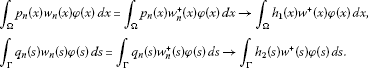Hence, ${ p n (x) w n (x)}$ is bounded in $L 2 (Ω)$, $p n (x) w n (x)⇀ h 1 (x) w + (x)$ in $L 2 (Ω)$; ${ q n (x) w n (x)}$ is bounded in $L 2 (Γ)$, $q n (x) w n (x)⇀ h 2 (x) w + (x)$ in $L 2 (Γ)$.

By (16), we have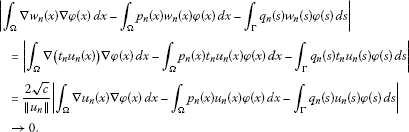Since $w n ⇀w$ is a weak convergence in V, we obtain

$∫ Ω ∇φ(x)∇w(x)dx− ∫ Ω h 1 (x)φ(x) w + (x)dx− ∫ Γ h 2 (s)φ(s) w + (s)ds=0,φ∈V.$

We order $φ= w −$; this yields $∥ w − ∥ 2 =0$, so $w= w + ≥0$. By the Strong maximum principle, we know $w>0$ a.e. in Ω, so $u n →∞$ a.e. in Ω. Combining (S3) and (3), we obtain

$∫ Ω ∇φ(x)∇w(x)dx− ∫ Ω q(x)φ(x)w(x)dx− ∫ Γ q(s)φ(s)w(s)ds=0,∀φ∈V.$

This is a contradiction with $Λ<1$. This completes the proof of Theorem 1(ii).

1. (iii)

If $Λ=1$, by Lemma 1, there exists some $φ Λ (x)>0$, such that

$∫ Ω ∇v(x)∇ φ Λ (x)dx= ∫ Ω q(x)v(x) φ Λ (x)dx+ ∫ Γ q(s)v(s) φ Λ (s)ds.$
(17)

If u is a positive solution of (1), for the above $φ Λ (x)$, we have

$∫ Ω ∇u(x)∇ φ Λ (x)dx= ∫ Ω f ( x , u ( x ) ) φ Λ (x)dx+ ∫ Γ g ( s , u ( s ) ) φ Λ (s)ds.$
(18)

We order $v=u$ in (17), and it follows from (18) that

$∫ Ω ∇ u ( x ) ∇ φ Λ ( x ) d x = ∫ Ω q ( x ) u ( x ) φ Λ ( x ) d x + ∫ Γ q ( s ) u ( s ) φ Λ ( s ) d s = ∫ Ω f ( x , u ( x ) ) φ Λ ( x ) d x + ∫ Γ g ( s , u ( s ) ) φ Λ ( s ) d s ≤ ∫ Ω q ( x ) u ( x ) φ Λ ( x ) d x + ∫ Γ q ( s ) u ( s ) φ Λ ( s ) d s ,$

which implies that $∫ Ω (f(x,u)−q(x)u(x)) φ Λ (x)dx+ ∫ Γ (g(s,u)−q(s)u(s)) φ Λ (s)ds=0$.

When $φ Λ (x)>0$ a.e. in Ω, combining (S2), (S3), and (3), we obtain

$f(x,u)≤q(x)u(x),g(x,u)≤q(x)u(x).$

Then we must have $f(x,u)=q(x)u(x)$, $g(x,u)=q(x)u(x)$ a.e. in Ω, $u(x)>0$ also achieves Λ (=1). When $u=c φ Λ$, $c>0$, we have $∫ Ω | ∇ φ Λ | 2 dx= ∫ Ω q(x) φ Λ 2 dx+ ∫ Γ q(s) φ Λ 2 ds$, which achieves Λ.

On the other hand, if for some $c>0$, $u(x)=c φ Λ (x)$ and $f(x,c φ Λ (x))=cq(x) φ Λ (x)$, $g(x,u)=cq(x) φ Λ (x)$ a.e. $x∈Ω$, since $c φ Λ (x)$ also achieves Λ. This means $u(x)=c φ Λ (x)$ is a solution of problem (1) as $Λ=1$. This completes the proof of Theorem 1(iii). □

Proof of Corollary 2 Note that when $q(x)≡l$, then $Λ= λ 1 l$. The conclusion follows from Theorem 1. □

Proof of Theorem 3 When $q(x)≡+∞$, we can replace $φ Λ$ by $φ 1$ in (11) and define c as in (12), then following the same procedures as in the proof of Theorem 1(ii), we need to show only that ${ u n }$ is bounded in V. For this purpose, let ${ w n }$ be defined as in (16). If ${ w n }$ is bounded in V, we know $w n →w$ is a strong convergence in $L 2 (Ω)$, $w n →w$ is convergence a.e. $x∈Ω$, $w n ⇀w$ is a weak convergence in V, and $w∈V$.

If $∥ u n ∥→+∞$, then $t n →0$ and $w(x)≡0$. We set $Ω 1 ={x∈Ω:w(x)=0}$, $Ω 2 ={x∈Ω:w(x)≠0}$. Obviously, by (16), $| u n |→+∞$ a.e. in $Ω 2$. When $q(x)≡+∞$ in (S3), there exists $K 1 , K 2 >0$ and n large enough we have $| f ( x , u n ) u n |≥ K 1$, $| g ( x , u n ) u n |≥ K 2$ uniformly in $x∈ Ω 2$. Hence, by (15) and (16), we obtain

$4 c = lim n → + ∞ t n 2 ∥ u n ∥ 2 = lim n → + ∞ t n 2 ( ∥ ∇ u n ∥ L 2 ( Ω ) 2 + ∥ γ u n ∥ L 2 ( Γ ) 2 ) = lim n → + ∞ t n 2 ( ∫ Ω f ( x , u n ) u n d x + ∫ Γ g ( s , u n ) u n d s + ∥ γ u n ∥ L 2 ( Γ ) 2 ) = lim n → + ∞ ( ∫ Ω f ( x , u n ) u n w n 2 d x + ∫ Γ g ( s , u n ) u n w n 2 d s + t n 2 ∥ γ u n ∥ L 2 ( Γ ) 2 ) ≥ K 1 ∫ Ω w 2 d x + K 2 ∫ Γ w 2 d s + ∥ w ∥ L 2 ( Γ ) 2 .$

Noticing that $w(x)≠0$ in $Ω 2$ and $K 1$, $K 2$ can be chosen large enough, so $m Ω 2 ≡0$ and then $w(x)≡0$ in Ω.

Then we know $lim n → + ∞ ∫ Ω F(x, w n )dx+ lim n → + ∞ ∫ Γ G(s, w n )ds=0$, and consequently,

$J ( w n ) = 1 2 ∥ ∇ w n ∥ L 2 ( Ω ) 2 + o ( 1 ) = 1 2 ∥ w n ∥ 2 − 1 2 ∥ w n ∥ L 2 ( Γ ) 2 + o ( 1 ) ≥ 1 2 ( 1 − 1 λ 1 + 1 ) ∥ w n ∥ 2 + o ( 1 ) = 2 c ( 1 − 1 λ 1 + 1 ) + o ( 1 ) .$
(19)

By $∥ u n ∥→+∞$, $t n →0$ as $n→+∞$, then it follows Lemma 4 and (13), we obtain

$J( w n )=J( t n u n )≤ 1 + t n 2 2 n ≤c.$
(20)

Obviously, (19) and (20) are contradictory. So ${ u n }$ is bounded in V. This completes the proof of Theorem 3. □

## 4 Example

In this section, we give two examples on $f(x,u)$: One satisfies (S1) to (S3) with $q(x)≡+∞$, but does not satisfy the (AR) condition; the other illustrates how the assumptions on the boundary are not trivial and compatible with the inner assumptions in Ω.

Example 1 Set:

$f(x,t)={ 0 , t ≤ 0 ; t l n ( 1 + t ) , t > 0 .$

Then it is easy to verify that $f(x,t)$ satisfies (S1) to (S3) with $p(x)=0$ as $t→0$ and $q(x)=+∞$ as $t→+∞$. In addition,

$F(x,t)= 1 2 t 2 ln(1+t)− 1 4 t 2 + 1 2 t− 1 2 ln(1+t).$

So, for some $μ>2$, $μF(x,t)= t 2 ln(1+t)( μ 2 − μ 4 ln ( 1 + t ) + μ 2 t l n ( 1 + t ) − μ 2 t 2 )> t 2 ln(1+t)$, for all t large.

This means $f(x,t)$ does not satisfy the (AR) condition.

Example 2 Consider the following problem:

${ − u ″ ( x ) = α u ( x ) , 0 < x < l , u ( 0 ) = 0 , u ′ ( l ) = α u ( l ) ,$
(21)

where $α>0$ is a constant. It is obvious that $g=γf$ as $f(x,u)=αu(x)$. Problem (21) is a case of (1); we can obtain the nontrivial solution: $u(x)= C ˜ sin α x$, $C ˜ ≠0$.

## References

1. 1.

Liu H, Su N: Well-posedness for a class of mixed problem of wave equations. Nonlinear Anal. 2009, 71: 17-27. doi:10.1016/j.na.2008.10.027

2. 2.

Landesman EM, Lazer AC: Nonlinear perturbations of linear elliptic boundary value problems at resonance. J. Math. Mech. 1970, 19: 609-623.

3. 3.

Ahmad S, Lazer AC, Paul JL: Elementary critical point theory and perturbations of elliptic boundary value problems at resonance. Indiana Univ. Math. J. 1976, 25: 933-944. 10.1512/iumj.1976.25.25074

4. 4.

Berestycki H, De Figueiredo DG: Double resonance in semilinear elliptic problems. Commun. Partial Differ. Equ. 1981, 6: 91-120. 10.1080/03605308108820172

5. 5.

Costa DG, Oliveira AS: Existence of solution for a class of semilinear elliptic problems at double resonance. Bol. Soc. Bras. Mat. 1988, 19: 21-37. 10.1007/BF02584819

6. 6.

Omari P, Zanolin F: Resonance at two consecutive eigenvalues for semilinear elliptic equations. Ann. Mat. Pura Appl. 1993, 163: 181-198. 10.1007/BF01759021

7. 7.

Binding PA, Drábek P, Huang YX: Existence of multiple solutions of critical quasilinear elliptic Neumann problems. Nonlinear Anal. 2000, 42: 613-629. doi:10.1016/S0362-546X(99)00118-2 10.1016/S0362-546X(99)00118-2

8. 8.

Li G, Zhou HS: Asymptotically linear Dirichlet problem for the p -Laplacian. Nonlinear Anal. 2001, 43: 1043-1055. doi:10.1016/S0362-546X(99)00243-6 10.1016/S0362-546X(99)00243-6

9. 9.

Escobar JF: A comparison theorem for the first non-zero Steklov eigenvalue. J. Funct. Anal. 2000, 178: 143-155. doi:10.1006/jfan.2000.3662 10.1006/jfan.2000.3662

10. 10.

Kaur BS, Sreenadh K: Multiple positive solutions for a singular elliptic equation with Neumann boundary condition in two dimensions. Electron. J. Differ. Equ. 2009, 43: 1-13.

11. 11.

Ahmad S: Multiple nontrivial solutions of resonant and nonresonant asymptoticaly problems. Proc. Am. Math. Soc. 1986, 96: 405-409. 10.1090/S0002-9939-1986-0822429-2

12. 12.

Chang KC: Infinite Dimensional Morse Theory and Multiple Solutions Problems. Birkhäuser, Boston; 1993.

13. 13.

Hirano N, Nishimura T: Multiplicity results for semilinear elliptic problems at resonance and with jumping nonlinearities. J. Math. Anal. Appl. 1993, 180: 566-586. doi:10.1006/jmaa.1993.1417 10.1006/jmaa.1993.1417

14. 14.

Landesman E, Robinson S, Rumbos A: Multiple solution of semilinear elliptic problem at resonance. Nonlinear Anal. 1995, 24: 1049-1059. doi:10.1016/0362-546X(94)00107-S 10.1016/0362-546X(94)00107-S

15. 15.

Liu SQ, Tang CL, Wu XP: Multiplicity of nontrivial solutions of semilinear elliptic equations. J. Math. Anal. Appl. 2000, 249: 289-299. doi:10.1006/jmaa.2000.6704 10.1006/jmaa.2000.6704

16. 16.

Li S, Willem M: Multiple solution for asymptotically linear boundary value problems in which the nonlinearity crosses at least one eigenvalue. Nonlinear Differ. Equ. Appl. 1998, 5: 479-490. 10.1007/s000300050058

17. 17.

Mawhin J, Willem M: Critical Point Theory and Hamiltonien Systems. Springer, New York; 1989.

18. 18.

Lions JL, Magenes E: Non-Homogeneous Boundary Value Problems and Applications. Springer, Berlin; 1972.

19. 19.

Zhou H: An application of a mountain pass theorem. Acta Math. Sin. Engl. Ser. 2002, 18(1):27-36. 10.1007/s101140100147

20. 20.

Cheng BT, Wu X: Existence results of positive solutions of Kirchhoff type problems. Nonlinear Anal. 2009, 71: 4883-4892. doi:10.1016/j.na.2009.03.065 10.1016/j.na.2009.03.065

21. 21.

Ambrosetti A, Rabinowitz PH: Dual variational methods in critical points theory and applications. J. Funct. Anal. 1973, 14: 349-381. doi:10.1016/0022-1236(73)90051-7 10.1016/0022-1236(73)90051-7

## Author information

Authors

### Corresponding author

Correspondence to Guofa Li.

### Competing interests

The author declares that he has no competing interests.

## Rights and permissions

Reprints and Permissions

Li, G. Existence of positive solutions of elliptic mixed boundary value problem. Bound Value Probl 2012, 91 (2012). https://doi.org/10.1186/1687-2770-2012-91